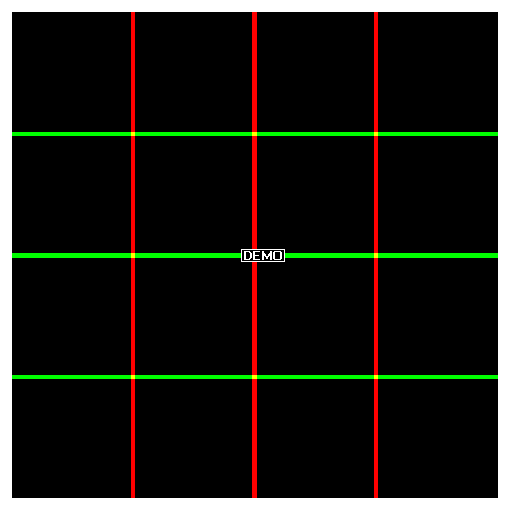Example 8.  Synergy and Antagonism for Colors in Diffusion

In this example, diffusion is simulated by the recursive use of a 10 x 10 averaging kernel to cause three vertical red lines and three horizontal green lines to diffuse into each other and into a 512 x 512 black background.  At the end of each of these 15 cycles of convolution, nine different decision algorithms (DAs) are used to evaluate red (R) and green (G) values at the nine intersections that are undergoing diffusion.  If the DAs find any pixels in their vicinity that cause the respective DA to be evaluated as 'true', then R and G are set to zero and blue (B) is set to 1. The resulting blue overlays demonstrate the principles of additivity, antagonism, and synergy that are frequently seen in applications related to antibiotic and antiviral pharmaceuticals.

The first image in the looped animated gif (8.1, below) shows only the three red and three green lines with no diffusion or overlay. The next 15 images show decreasing values of the red and green values at the former positions of sharp lines as the convolution kernel (100 elements, all valued 0.01) causes red and green color diffusion.  The initial lines have a finite width of six pixels set either to R=1 or G=1.  All other pixels are within a black (K) background of R=G=B=0 into which the red and green lines diffuse.  The blue overlay is not allowed to diffuse; it is evaluated on a per cycle basis.There are nine decisions algorithms DAs that evaluate the red (R) and green (G) channels to determine whether (if true) the blue (B) channel will be turned on (B=1) and R and G will both be turned off (R=G=0).  Each DA is evaluated only in the vicinity of a single intersection as specified by the positions stated in the first column of the table below:

Top Left                Combined by OR                  R > 0.3 || G > 0.3

Top Center            Combined by addition           R + G > 0.3

Top Right               OR, asymmetric threshold     R > 0.4 || G > 0.2

Center Left             Antagonism                          ((R > 0.2) && (G <0.1)) || ((G>0.2) && (R <0.1))

Center                    Stronger antagonism             ((R > 0.2) && (G <0.01)) || ((G > 0.2) && (R < 0.01))

Right Center           Asymmetric antagonism         ((R > 0.2) && (G < 0.001)) || ((G > 0.2) && (R < 0.1))

Bottom Left            Synergy                                R * G > 0.01

Bottom Center        Synergy with threshold          0.01 > R * G  > 0.0001

Bottom Right          Asymmetric synergy              R * G^2 >0.001

Key:  Logical OR || ; logical AND && ; addition + ; greater than > ; less than < ; multiply * ; exponent ^ ; ( ) nested order.

The numbers shown in the table, above, can be considered as analogous to minimal inhibitory concentrations (MICs) of antibiotics, and the blue regions in the image can be considered as analogous to clearing zones in a bacterial lawn.  Antibiotic synergy is indeed well known in the scientific literature.  Synergist drugs have the advantage that very low concentrations of two drugs can be used rather than a higher concentration of either single drug.  In an actual physical embodiment of this method, the DA is a cellular pathway, and 'true' means death (clearing) of the bacterial lawn.  In other analogies, arrayed chemicals that interact favorably by synergy or unfavorably by antagonism can be assayed by arraying them in such an interaction grid and assaying for the desired reaction.  A grid of 100 x100 lines (i.e., 100 different chemicals) yields 10,000 interactions with 100 homologous reactions on the diagonal and (10,000-100)/ 2= 4,450 unique interactions duplicated above and below the diagonal.

Source code is given here in .nb and .PDF format.  The PDF format contains all 16 frames of the diffusion images used an the animation, and it shows much greater detail than the animated gif on this webpage.